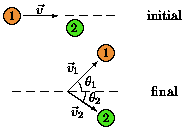# Basics True or False, Momentum Problem

NoobeAtPhysics

## Homework StatementThis problem has analogies in the game of pool. Puck 1 slides on a horizontal, frictionless surface with velocity v and collides elastically with stationary puck 2; each puck has the same mass, m. The pucks move off with velocities v1 and v2, as shown. The angles θ1 and θ2 are measured relative to the initial direction of puck 1.

Which of these statements is correct?

1) v^2 = v1^2+v2^2
2) This problem has analogies to pool because pool balls have equal mass and roll without friction. [As long as rotational KE of pool balls is neglected, the analogy is perfect.]
3) In this problem, linear momentum is conserved but kinetic energy is not conserved.
4) 0 = v1sinθ1−v2sinθ2
5) If b+c = a for three non-zero vectors, and b^2+c^2 = a^2, then the angle between a and c is 90°.

## Homework Equations

mv1 + mv2 = mv1' + mv2'

## The Attempt at a Solution

1) False
2) True
3) False
4) False
5) True

Thinking why Im wrong here, maybe it is number 1? Using the momentum formula, I find that v1 = v1' + v2', not the equation described.

best regards,
Noobe

Last edited:

MGCLO
funny how everyone is looking for an answer. not to answer someone help. people are very greedy. or at least most of them. I never took the stuff above so I cant answer

NoobeAtPhysics
Sorry I would help you but i'm still learning first year physics

NoobeAtPhysics
maybe i should explain further,

1) False, because v = v1 + v2 because of conservation of momentum

2) True, because it makes sense that a pool table would be similar and even says in the question

3) False, linear momentum is of course conserved because of the conservation of momentum

4) False, they go off at completely different velocities right? even in y direction

5) True, The picture is at a 90 degree angle and I read somewhere that this is true, because of right triangles and c and b are the legs of the triangle

Last edited:
nil1996
Why do you think kinetic energy is not conserved??

NoobeAtPhysics
oh woops, typo

NoobeAtPhysics
still looks wrong guys. maybe wat is going on

nil1996
Kinetic energy and linear momentum both are conserved in elastic collisions.

NoobeAtPhysics
I heard that was true about energy but maybe not momentum,?

also, the question asks one or the other right? one has to be conserved and the other cannot be conserved. I want to say that kinetic energy is defentiely conserved because where else does the energy go right.

NoobeAtPhysics
please let me know if anyone understands

Last edited by a moderator:
nil1996
if that was not an elastic collision then Kinetic energy would not have been conserved because there will be energy loses in form of sound energy,change in shape of ball,heat energy, etc

willem2
You should try to actually compute a few answers. You can compute everything there is to compute with conservation of energy and conservation of momentum (both in the x and y direction)

1) Since the collision is elastic, kinetic energy is conserved. If you would write down what that means, it would be easy to see the right answer.

2) ok

3) ok, but you seem to have misread the question, because the part about energy conservation is wrong.

4) try to compute this.

5) "because of right triangles" isn't very sound reasoning. Have you heard of the cosine rule?

NoobeAtPhysics
dont worry dudes, i just asked my teacher and he told me the answers